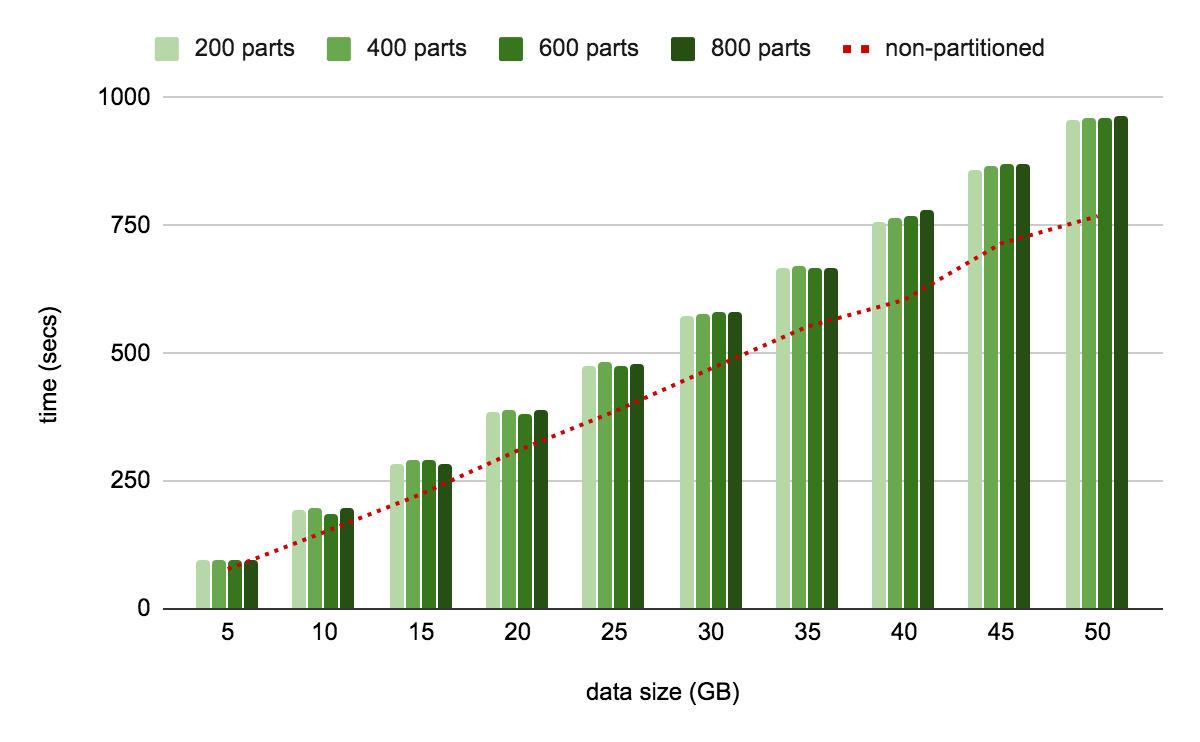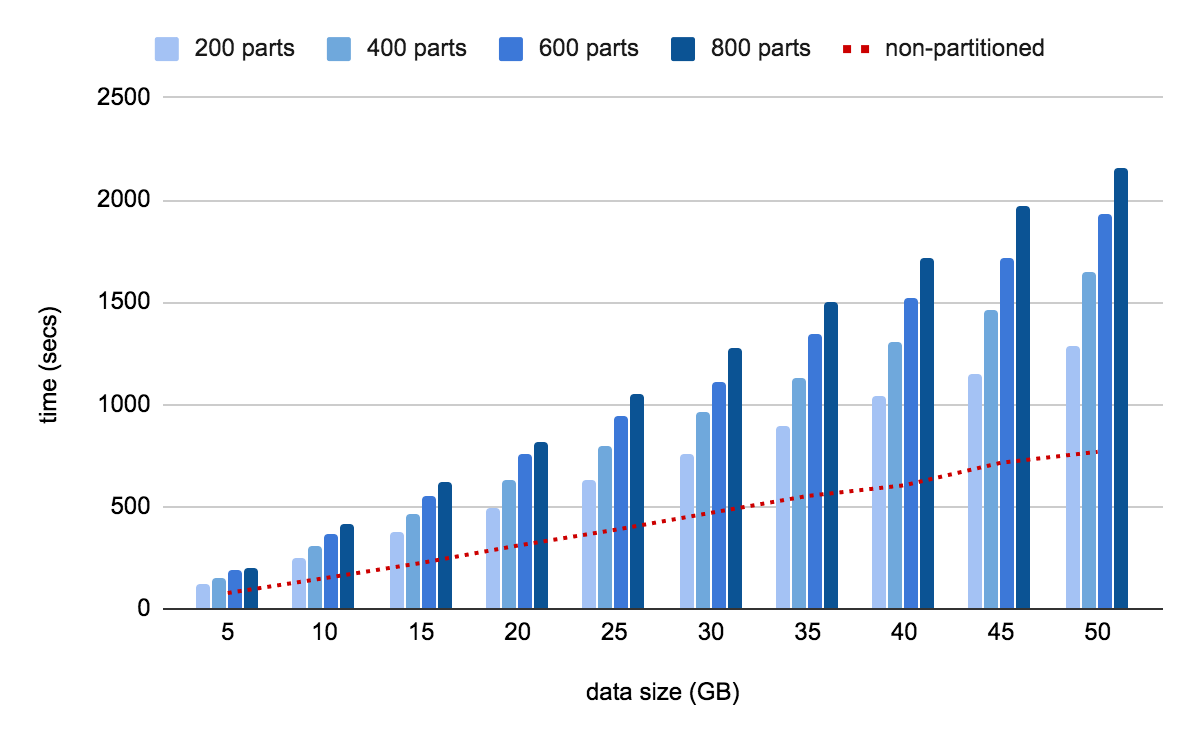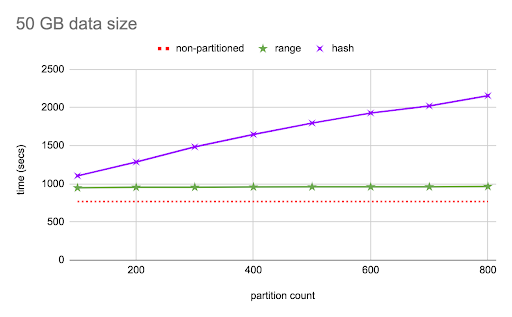SUMMARY: This article covers how benchmark tests can be used to demonstrate the effect of table partitioning on performance. Tests using range- and hash-partitioned tables are compared and the reasons for their different results are explained:

1. Range partitions

2. Hash partitions

3. Combination graphs

4. Explaining the behavior

5. Conclusion

Partitioning was introduced in PostgreSQL 10 to increase performance in certain scenarios. In PostgreSQL 13, pgbench was extended to run benchmark tests for range and hash partitions by partitioning the pgbench_accounts table according to the parameters specified. Since pgbench_accounts is the largest table, the bulkload command COPY is used to populate it. This post will explore how this operation is affected by table partitioning.

Tests were performed for partition counts of 200, 400, 600, and 800 on pgbench scales varying from 500 (5 GB) to 5000 (50GB) for both the range and hash partition types with the following settings:

• pgbench thread/client count = 32
• shared_buffers = 32GB
• min_wal_size = 15GB
• max_wal_size = 20GB
• checkpoint_timeout=900
• maintenance_work_mem=1GB
• checkpoint_completion_target=0.9
• synchronous_commit=on

The hardware specification of the machine on which the benchmarking was performed is as follows:

• IBM POWER8 Server
• Red Hat Enterprise Linux Server release 7.1 (Maipo) (with kernel Linux 3.10.0-229.14.1.ael7b.ppc64le)
• 491GB RAM
• IBM,8286-42A CPUs (24 cores, 192 with HT)

## Range partitionsThe red dotted lines in this chart show the performance for an unpartitioned table across different data sizes. It is evident that the range-partitioned table takes a slightly longer time, but increasing the partition count hardly influences the load time.

## Hash partitionsThe amount of time taken by a hash-partitioned table with the lowest partition count and lowest data size (200 parts, 5GB) is 60% more than that of the unpartitioned table, and the time taken for the hash-partitioned table with the highest partition count and largest data size (800 parts, 50 GB) is 180% more than that of the unpartitioned table. It is obvious that the number of partitions has heavily impacted the load time.

## Combination graphs

Here are two graphs that merge the results of the range and hash partitions to distinctly show the difference between the two types.This first graph displays how range and hash partitioned tables with 400 partitions compare against a non-partitioned table for different data sizes. In all cases, there is a general upward trend as the data size to be loaded increases, which is expected—larger the data, the longer the time taken. The range-partitioned table takes about 20–25% more time than the unpartitioned table, and the hash partition shows a steep substantial, increase in load time as the data size increases.This second graph presents how the range and hash partitioned tables compare against a non-partitioned table for different partition counts at a data size of 50 GB. Here again, the range partition show a steady 20–25% increase, but the hash partitioned table exhibits a more dramatic change as the partition count increases.

## Explaining the behavior

Though size plays an expected influence on the load time, the hash partitioned table is also profoundly impacted by the number of partitions the table has.

To understand this, let us look at how pgbench partitions and inserts data into the table. The pgbench_accounts table is partitioned on the aid column, which is called the partition key, and a series of data for aid values are generated in sequence (from 1 to pgbench_scale * 100000) and inserted using the COPY command. In COPY the tuple routing parameters are set for the partitioned table, and then the data is inserted. The range-partitioned table splits data based on the data value such that each partition can hold all tuples with aid values that fall within the range, and the hash-partitioned table uses a modulo operator on the value being inserted, and the remainder obtained by performing the operation is used to determine the partition into which that value will be inserted.

Since pgbench copies data in order according to the partition key (aid column), the range partition insertion behaviour shows an ideal case where data of one partition is fully inserted before moving on to the next partition. For the hash-partitioned table, the first value is inserted in the first partition, the second number in the second partition and so on till all the partitions are reached before it loops back to the first partition again until all the data is exhausted. Thus it exhibits the worst-case scenario where the partition is repeatedly switched for every value inserted. As a result, the number of times the partition is switched in a range-partitioned table is equal to the number of partitions, while in a hash-partitioned table, the number of times the partition has switched is equal to the amount of data being inserted. This causes the massive difference in timing for the two partition types.

## Conclusion

When using COPY for data ordered on the partition key column, no matter the size or the number of partitions, the operation would take about 20–25% more time than an unpartitioned table. If the data being copied is unordered with respect to the partition key then the time taken will depend on how often the partition has to be switched between insertions.

To speed up a COPY operation, it is advisable to have data sorted according to the partition key of the table so that there is minimal partition switching.